UIHistories Project: A History of the University of Illinois by Kalev Leetaru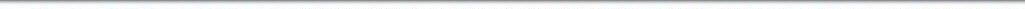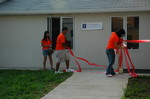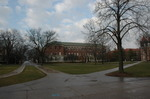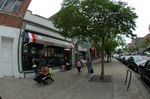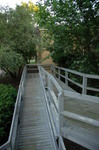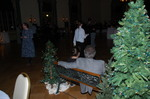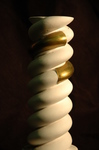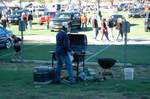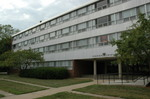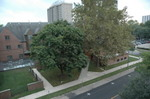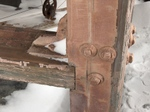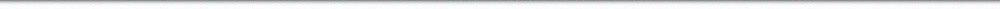N A V I G A T I O N D I G I T A L L I B R A R Y

## Repository: UIHistories Project: Board of Trustees Minutes - 1896 [PAGE 166]

Caption: Board of Trustees Minutes - 1896
This is a reduced-resolution page image for fast online browsing.

< Previous Page [Displaying Page 166 of 371] Next Page >
[VIEW ALL PAGE THUMBNAILS]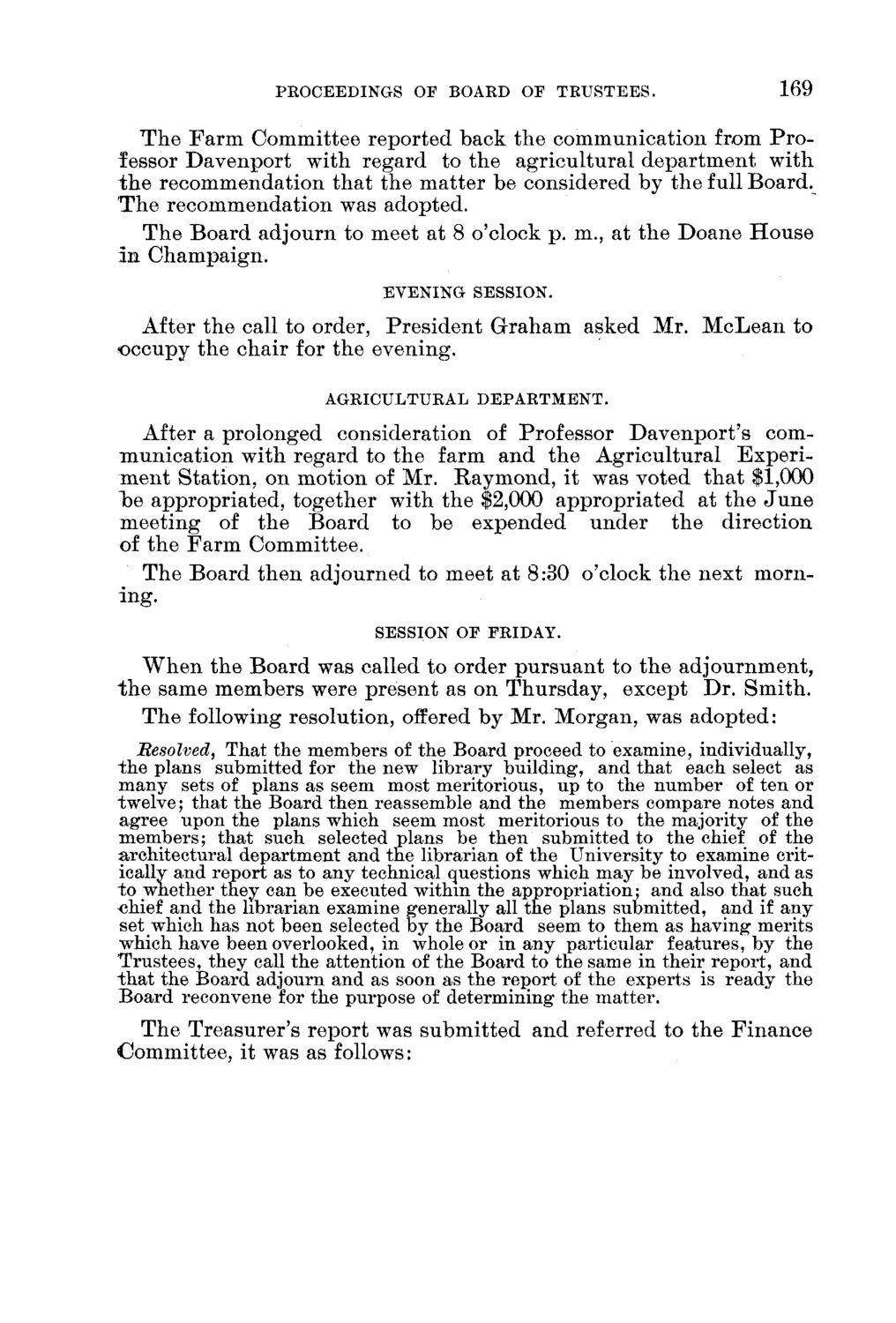### EXTRACTED TEXT FROM PAGE:

PROCEEDINGS OF BOARD OF TRUSTEES.

169

T h e F a r m C o m m i t t e e r e p o r t e d b a c k t h e c o m m u n i c a t i o n from P r o fessor D a v e n p o r t w i t h r e g a r d to t h e a g r i c u l t u r a l d e p a r t m e n t w i t h t h e r e c o m m e n d a t i o n t h a t t h e m a t t e r be considered by t h e full B o a r d . T h e r e c o m m e n d a t i o n was adopted. T h e B o a r d a d j o u r n to meet at 8 o'clock p . m., at t h e D o a n e H o u s e In Champaign.

EVENING SESSION.

After t h e call to order, P r e s i d e n t G r a h a m asked Mr. M c L e a n to o c c u p y t h e c h a i r for t h e e v e n i n g .

AGRICULTURAL DEPARTMENT.

After a prolonged consideration of Professor D a v e n p o r t ' s comm u n i c a t i o n w i t h r e g a r d to t h e farm a n d t h e A g r i c u l t u r a l E x p e r i m e n t S t a t i o n , on m o t i o n of Mr. R a y m o n d , it was voted t h a t \$1,000 lye a p p r o p r i a t e d , t o g e t h e r with t h e \$2,000 a p p r o p r i a t e d at t h e J u n e m e e t i n g of t h e B o a r d to be e x p e n d e d u n d e r t h e d i r e c t i o n of t h e F a r m C o m m i t t e e . T h e B o a r d t h e n a d j o u r n e d to meet at 8:30 o'clock t h e n e x t m o r n ing.

SESSION OF FRIDAY.

W h e n t h e B o a r d was called to order p u r s u a n t to t h e a d j o u r n m e n t , t h e same m e m b e r s were p r e s e n t as on T h u r s d a y , except D r . S m i t h . T h e following resolution, offered b y Mr. M o r g a n , was a d o p t e d : Resolved, That the members of the Board proceed to examine, individually, the plans submitted for the new library building", and that each select as many sets of plans as seem most meritorious, up to the number of ten or twelve; that the Board then reassemble and the members compare notes and agree upon the plans which seem most meritorious to the majority of the members; that such selected plans be then submitted to the chief of the architectural department and the librarian of the University to examine critically and report as to any technical questions which may be involved, and as to whether they can be executed within the appropriation; and also that such chief and the librarian examine generally all the plans submitted, and if any set which has not been selected by the Board seem to them as having merits which have been overlooked, in whole or in any particular features, by the Trustees, they call the attention of the Board to the same in their report, and that the Board adjourn and as soon as the report of the experts is ready the Board reconvene for the purpose of determining the matter. T h e T r e a s u r e r ' s r e p o r t was s u b m i t t e d a n d referred to t h e F i n a n c e C o m m i t t e e , it was as follows: